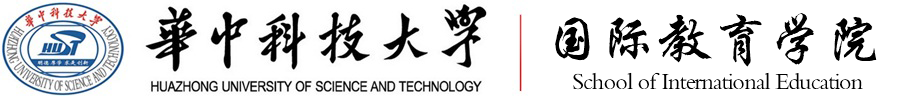﻿ Wang Xiangjun-International Student Office, HUSTName: Wang Xiangjun Professor in School of Mathematics and Statistics Phone: 86-27-18971429466 Email: xjwang@hust.edu.cn Academic Areas: Department of Probability and Statistics

Research Interest

Stochastic Anlaysis; Statistics

Education

Doctor--Probability Theory and Mathematical Statistics--Huazhong University of Science and Technology, Wuhan,China--1999

Master--Probability Theory and Mathematical Statistics--Wuhan University, Wuhan,China--1996

Bachelor--Class of Sino-Frech--Wuhan University, Wuhan,China--1993

Honours and Awards

 X. J. Wang, J. H. Wen, X. P. Xiao,Grey parametric for small sample observations of stochastic differential equations,J. Grey Sys,2012,

 XiangjunWanga, GuoJiang,Lp-strong solutions of stochastic partial differential equations with monotonic drifts,JMAA,2014,

 AL-Hashimi, Muzahem M. Y., and X. J. Wang, Trend of leukemia in Ninawa/Iraq ,Clinical and Experimental Medical Sciences,2013,

 G. Jiang, X. J. Wang,Regularity property of solution to two-parameter stochastic Volterra equation with non-Lipschitz coefficients,Acta Mathematica Scientia,2013,

 X. J. Wang, J. H. Wen, J. P. Li, J. Q. Duan,Impact of α-stable Levy noise on the Stommel model for the thermohaline circulation,DCDS-B,2012,

 X. J. Wang, J. J. Guo, G. Jiang,Collision local times of two independent fractional Brownian motions,Front. Math. China,2011,